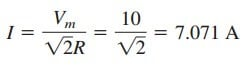# Complete Series Resonance Circuit FormulaThe most prominent feature of the frequency response of a circuit may be the sharp peak (or resonant peak) exhibited in its amplitude characteristic. The concept of resonance applies in several areas of science and engineering.

Contents

Resonance occurs in any system that has a complex conjugate pair of poles; it is the cause of oscillations of stored energy from one form to another.

## Series Resonance

It is the phenomenon that allows frequency discrimination in communications networks. Resonance occurs in any circuit that has at least one inductor and one capacitor.

Resonance is a condition in an RLC circuit in which the capacitive and inductive reactances are equal in magnitude, thereby resulting in a purely resistive impedance.

Series or parallel resonant circuits are useful for constructing filters, as their transfer functions can be highly frequency-selective. They are used in many applications such as selecting the desired stations in radio and TV receivers.

Consider the series RLC circuit shown in Figure.(1) in the frequency domain. The input impedance is

or

Resonance results when the imaginary part of the transfer function is zero, or

The value of ω that satisfies this condition is called the resonant frequency ω0. Thus, the resonance condition is

or

Since ω0 = 2πf0,

Note that at resonance:

1. The impedance is purely resistive, thus, Z = R. In other words, the LC series combination acts like a short circuit, and the entire voltage is across R.
2. The voltage Vs and the current I are in phase, so that the power factor is unity.
3. The magnitude of the transfer function H(ω) = Z(ω) is minimum.
4. The inductor voltage and capacitor voltage can be much more than the source voltage.

The frequency response of the circuit’s current magnitude

is shown in Figure.(2); the plot only shows the symmetry illustrated in this graph when the frequency axis is a logarithm.

The average power dissipated by the RLC circuit is

The highest power dissipated occurs at resonance, when I = Vm/R, so that

At certain frequencies ω = ω1, ω2, the dissipated power is half the maximum value; that is,

Hence, ω1 and ω2 are called the half-power frequencies.

The half-power frequencies are obtained by setting Z equal to2R, and writing

Solving for ω, we obtain

We can relate the half-power frequencies with the resonant frequency. From Equations.(5) and (12),

showing that the resonant frequency is the geometric mean of the half-power frequencies. Notice that ω1 and ω2 are in general not symmetrical around the resonant frequency ω0, because the frequency response is not generally symmetrical.

However, as will be explained shortly, the symmetry of the half-power frequencies around the resonant frequency is often a reasonable approximation.

Although the height of the curve in Figure.(2) is determined by R, the width of the curve depends on other factors. The width of the response curve depends on the bandwidth B, which is defined as the difference between the two half-power frequencies,

This definition of bandwidth is just one of several that are commonly used. Strictly speaking, B in Equation.(14) is a half-power bandwidth, because it is the width of the frequency band between the half-power frequencies.

The “sharpness” of the resonance in a resonant circuit is measured quantitatively by the quality factor Q. At resonance, the reactive energy
in the circuit oscillates between the inductor and the capacitor. The quality factor relates the maximum or peak energy stored to the energy dissipated in the circuit per cycle of oscillation:

It is also regarded as a measure of the energy storage property of a circuit in relation to its energy dissipation property. In the series RLC circuit, the peak energy stored is ½LI2, while the energy dissipated in one period is ½(I2R)(1/f ). Hence,

or

Notice that the quality factor is dimensionless. The relationship between
the bandwidth
B and the quality factor Q is obtained by substituting Equation.(12) into Equation.(14) and utilizing Equation.(17).

or B = ω02C R. Thus

The quality factor of a resonant circuit is the ratio of its resonant frequency to its bandwidth.

Keep in mind that Equations.(5), (12), (17), and (18) only apply to a series RLC circuit.

As illustrated in Figure.(3), the higher the value of Q, the more selective the circuit is but the smaller the bandwidth. The selectivity of an RLC circuit is the ability of the circuit to respond to a certain frequency and discriminate against all other frequencies.

If the band of frequencies to be selected or rejected is narrow, the quality factor of the resonant circuit must be high. If the band of frequencies is wide, the quality factor must be low.

A resonant circuit is designed to operate at or near its resonant frequency. It is said to be a high-Q circuit when its quality factor is equal to or greater than 10.

For high-Q circuits (Q 10), the half-power frequencies are, for all practical purposes, symmetrical around the resonant frequency and can be approximated as

High-Q circuits are used often in communications networks.

We see that a resonant circuit is characterized by five related parameters: the two half-power frequencies ω1 and ω2, the resonant frequency ω0, the bandwidth B, and the quality factor Q.

## Series Resonance Example

Let us try to analyze an RLC circuit below:

In the circuit in Figure.(4), R = 2 &, L = 1 mH, and C = 0.4 µF.

(a) Find the resonant frequency and the half-power frequencies. (b) Calculate the quality factor and bandwidth. (c) Determine the amplitude of the current at ω0, ω1, and ω2.

Solution:

(a) The resonant frequency is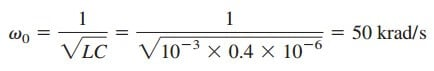METHOD 1:

The lower half-power frequency isSimilarly, the upper half-power frequency is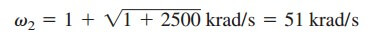(b) The bandwidth isor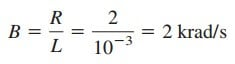The quality factor is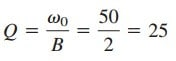METHOD 2

Alternatively, we could find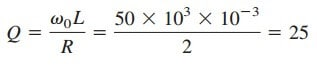From Q, we find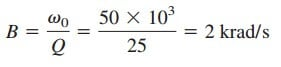Since Q > 10, this is a high-Q circuit and we can obtain the half-power frequencies asas obtained earlier.

(c) At ω = ω0,At ω = ω1, ω2,